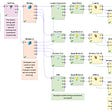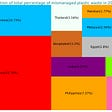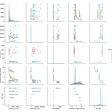# working with kolmogrov test

Hi, I am trying to use kolmogorov test which I’ going to use it in my artickle , I generate a data set A then I randomly made a sample set from A. then I wanated to compare these two sample sets with kstest. but It showed me they don’t have same distribution.

here is my simple code:

`clcclear allclose alln_s = 1000;mother_random_variable = lognrnd(0.3,0.5,[1,100000]);               %data lognormalS = mother_random_variable(randi(numel(mother_random_variable),1,n_s))          %sampleS_y = [S]';                             %selected data S_mean=mean(S_y);               %mean sampleS_var=std(S_y);                 %variance sammpletest_cdf = [S_y,cdf('Lognormal',S_y,S_var,S_mean)];        %make cdf kstest(S_y,'CDF',test_cdf)                  %ktestplot(sort(S_y),logncdf(sort(S_y)),'r--')hold oncdfplot(S_y)`

they have same distribution and ITs srange result . I found more strage result when I compare my data set with itself, Its result shows me they don’t have same distribution.

`clcclear allclose alln_s = 1000;mother_random_variable = lognrnd(0.3,0.5,[1,100000]); %dataS=mother_random_variable; % I named data with S for simpler codeS_y = [S]';     %selected data S_mean=mean(S_y);S_var=std(S_y);test_cdf = [S_y,cdf('Lognormal',S_y,S_var,S_mean)];kstest(S_y,'CDF',test_cdf)plot(sort(S_y),logncdf(sort(S_y)),'r--')hold oncdfplot(S_y)`

DO you have any Idea.

NOTE:-

Matlabsolutions.com provide latest MatLab Homework Help,MatLab Assignment Help for students, engineers and researchers in Multiple Branches like ECE, EEE, CSE, Mechanical, Civil with 100% output.Matlab Code for B.E, B.Tech,M.E,M.Tech, Ph.D. Scholars with 100% privacy guaranteed. Get MATLAB projects with source code for your learning and research.

Having only looked at your 2nd block of code, I have some comments and suggestions.

1) The parameters for a lognormal distribution are mean and standard deviation in that order. In your code, you’re entering them in reverse when you call the cdf() function and this is creating a totally different distribution than you intend to do.

`y = cdf('Lognormal', S_y, S_var, S_mean);    % your code, incorrecty = cdf('Lognormal', S_y, S_mean, S_var);    % correct`

2) This is just a suggestion but it’s a bit cleaner to use the makedist() function rather than entering the parameters manually into cdf().

`doc cdfpd = makedist('Lognormal', 'mu', S_mean, 'sigma', S_var); y = cdf(pd, S_y);   % instead of cdf('Lognormal', S_y, S_mean, S_var)`

3) “ when I compare my data set with itself, Its result shows me they don’t have same distribution.” But you aren’t comparing your data with itself. You’re comparing your data with the results of the cumulative distribution function of your data. The plot below shows the distribution of values from your data (top) and the distribution of values from the CDF. Clearly those distributions differ and the kstest() correctly rejects the null hypothesis.

--

--

--

## More from Technical Source

Simple! That is me, a simple person. I am passionate about knowledge and reading. That’s why I have decided to write and share a bit of my life and thoughts to.

Love podcasts or audiobooks? Learn on the go with our new app.

## Daily practices to optimize remote survey teams## Rule-Based Classification## Data is not the new oil! and why we’re building the Dataville ecosystem## Predicting the resilience of charter schools## Deep recommender systems for online recruitment## Decorators and Closures by Example in Python## Anomaly Detection using Rapidminer and Python## Technical Source

Simple! That is me, a simple person. I am passionate about knowledge and reading. That’s why I have decided to write and share a bit of my life and thoughts to.

## Nurturing a machine learning model## How to Create a Dataset for Machine Learning## Exploring Star Data Using Machine Learning